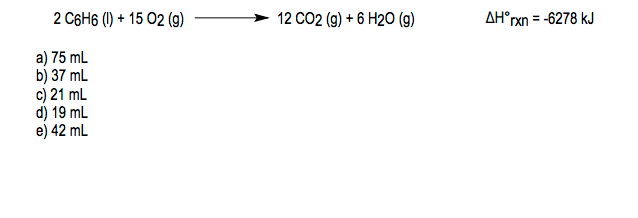# Problem: What volume of benzene (C6H6, d= 0.88 g/mL, molar mass = 78.11 g/mol) is required to produce 1.5 x 103 kJ of heat according to the following reaction?

###### FREE Expert Solution
90% (259 ratings)
###### Problem Details

What volume of benzene (C6H6, d= 0.88 g/mL, molar mass = 78.11 g/mol) is required to produce 1.5 x 103 kJ of heat according to the following reaction?What scientific concept do you need to know in order to solve this problem?

Our tutors have indicated that to solve this problem you will need to apply the Thermochemical Equations concept. You can view video lessons to learn Thermochemical Equations. Or if you need more Thermochemical Equations practice, you can also practice Thermochemical Equations practice problems.

What is the difficulty of this problem?

Our tutors rated the difficulty ofWhat volume of benzene (C6H6, d= 0.88 g/mL, molar mass = 78....as medium difficulty.

How long does this problem take to solve?

Our expert Chemistry tutor, Jules took 2 minutes and 30 seconds to solve this problem. You can follow their steps in the video explanation above.

What professor is this problem relevant for?

Based on our data, we think this problem is relevant for Professor Dunning's class at TEXAS.